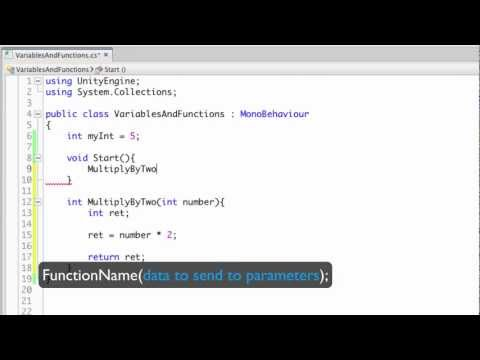# Variables and Functions

#### 난이도: 초급

What are Variables and Functions, and how do they store and process information for us?## Variables and Functions

초급 Scripting

### Code snippet

``````using UnityEngine;
using System.Collections;

public class VariablesAndFunctions : MonoBehaviour
{
int myInt = 5;

void Start ()
{
myInt = MultiplyByTwo(myInt);
Debug.Log (myInt);
}

int MultiplyByTwo (int number)
{
int ret;
ret = number * 2;
return ret;
}
}``````
``````#pragma strict

var myInt : int = 5;

function Start ()
{
myInt = MultiplyByTwo(myInt);
Debug.Log (myInt);
}

function MultiplyByTwo (number : int) : int
{
var ret : int;
ret = number * 2;
return ret;
}``````
``````import UnityEngine
import System.Collections

public class VariablesAndFunctions(MonoBehaviour):

private myInt = 5

private def Start():
myInt = MultiplyByTwo(myInt)
Debug.Log(myInt)

private def MultiplyByTwo(number as int) as int:
ret as int
ret = (number * 2)
return ret``````